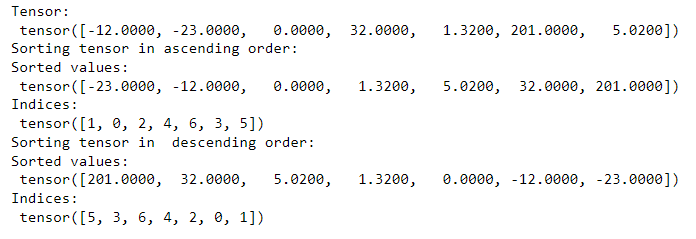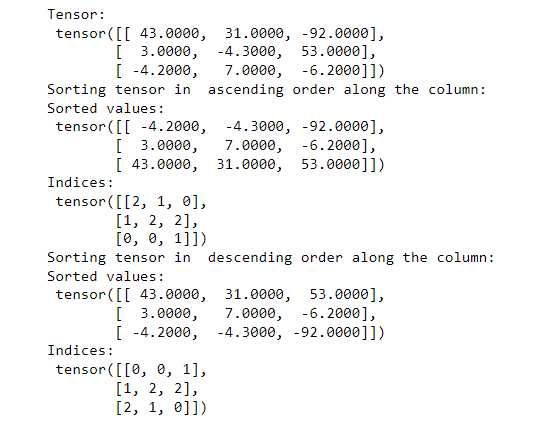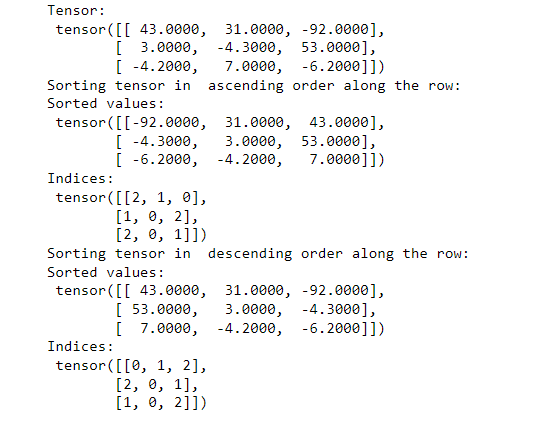Open in App
Not now

# How To Sort The Elements of a Tensor in PyTorch?

• Last Updated : 20 Feb, 2022

In this article, we are going to see how to sort the elements of a PyTorch Tensor in Python.

To sort the elements of a PyTorch tensor, we use torch.sort() method.  We can sort the elements along with columns or rows when the tensor is 2-dimensional.

Syntax: torch.sort(input, dim=- 1, descending=False)

Parameters:

• input: It is an input PyTorch tensor.
• dim: The dimension along which the tensor is sorted. It is an optional int value.
• descending: An optional boolean value used for sorting tensor elements in ascending or descending order. Default is set to False, sorting in ascending order.

Returns: It returns a named tuple of (values, indices), where values are the sorted values and indices are the indices of the elements in the original input tensor.

### Example 1:

In the below example, we sort the elements of a 1-dimensional tensor in ascending and descending orders. Sort tensor in ascending or descending order. We apply the torch.sort() method to sort the elements of an input tensor. To sort in descending order pass descending=True to the method.

## Python3

 `# importing required library``import` `torch`` ` `# defining a PyTorch Tensor``tensor ``=` `torch.tensor([``-``12``, ``-``23``, ``0.0``, ``32``,``                       ``1.32``, ``201``, ``5.02``])``print``(``"Tensor:\n"``, tensor)`` ` `# sorting the tensor in ascending order``print``(``"Sorting tensor in ascending order:"``)``values, indices ``=` `torch.sort(tensor)`` ` `# printing values of sorted tensor``print``(``"Sorted values:\n"``, values)`` ` `# printing indices of sorted value``print``(``"Indices:\n"``, indices)`` ` `# sorting the tensor in  descending order``print``(``"Sorting tensor in  descending order:"``)``values, indices ``=` `torch.sort(tensor, descending``=``True``)`` ` `# printing values of sorted tensor``print``(``"Sorted values:\n"``, values)`` ` `# printing indices of sorted value``print``(``"Indices:\n"``, indices)`

Output:### Example 2:

In this example, we sort the elements of a 2-dimensional tensor in ascending as well as descending orders along with the columns.

## Python3

 `# importing the library``import` `torch`` ` `# define a 2D torch tensor``tensor ``=` `torch.tensor([[``43``,``31``,``-``92``],``                       ``[``3``,``-``4.3``,``53``], ``                       ``[``-``4.2``,``7``,``-``6.2``]])``print``(``"Tensor:\n"``, tensor)`` ` `# sorting the tensor in  ascending order``print``("Sorting tensor ``in` `\``ascending order along the column:")``values, indices ``=` `torch.sort(tensor, dim ``=` `0``)`` ` `# printing  values in sorted tensor``print``(``"Sorted values:\n"``, values)`` ` `# print indices of values in sorted tensor``print``(``"Indices:\n"``, indices)`` ` `# sorting the tensor in  descending order``print``("Sorting tensor ``in` `\``descending order along the column:")``values, indices ``=` `torch.sort(tensor, dim ``=` `0``,``                             ``descending``=``True``)`` ` `# printing  values in sorted tensor``print``(``"Sorted values:\n"``, values)`` ` `# print indices of values in sorted tensor``print``(``"Indices:\n"``, indices)`

Output:### Example 3:

In this example, we sort the elements of a 2-dimensional tensor in ascending as well as descending orders along the rows.

## Python3

 `# importing the library``import` `torch`` ` `# define a 2D torch tensor``tensor ``=` `torch.tensor([[``43``, ``31``, ``-``92``], ``                       ``[``3``, ``-``4.3``, ``53``], ``                       ``[``-``4.2``, ``7``, ``-``6.2``]])``print``(``"Tensor:\n"``, tensor)`` ` `# sorting the tensor in  ascending order``print``("Sorting tensor ``in` `\``ascending order along the row:")``values, indices ``=` `torch.sort(tensor, dim``=``1``)`` ` `print``(``"Sorted values:\n"``, values)`` ` `# print indices of values in sorted tensor``print``(``"Indices:\n"``, indices)`` ` `# sorting the tensor in  descending order``print``("Sorting tensor ``in` `\``descending order along the row:")``values, indices ``=` `torch.sort(tensor,``                             ``dim``=``1``,``                             ``descending``=``True``)`` ` `# printing  values in sorted tensor``print``(``"Sorted values:\n"``, values)`` ` `# printing indices of values in sorted tensor``print``(``"Indices:\n"``, indices)`

Output:My Personal Notes arrow_drop_up